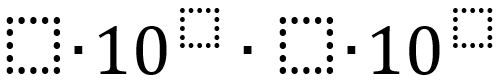# Scientific Notation

Directions: Using the digits 1 to 9 at most one time each, fill in the boxes to make the greatest (or least) product.### Hint

Where is the best place to put the biggest numbers?

Why should they go there?

7*10^9 * 6*10^8

Source: Bryan Meyer

## Systems of Equations 1

Directions: Using the integers -9 to 9 at most one time each, fill in the …

1.Smallest value?

(4*10^1)*(3*10^2)

2.how do I get this to equal 800,000,000

3.2 x 10^1 x 3 x 10^0

•digits 1-9 a 0 would not be allowed
Answer is: 4 times 10^1 times 3 times 10^2